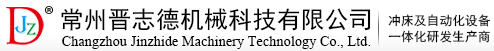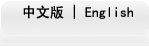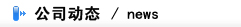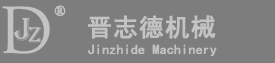温馨提示:-- var enabled = 0; today = new Date(); var day; var date; if(today.getDay()==0) day = "星期日" if(today.getDay()==1) day = "星期一" if(today.getDay()==2) day = "星期二" if(today.getDay()==3) day = "星期三" if(today.getDay()==4) day = "星期四" if(today.getDay()==5) day = "星期五" if(today.getDay()==6) day = "星期六" date1 = (today.getYear()) + "年" + (today.getMonth() + 1 ) + "月" + today.getDate() + "日" ; date2 = day ; document.write( date1 + date2 );

•现在位置：公司动态